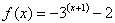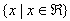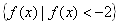Example 3

Find the domain and range of the function:.

Step 1. Find the domain.

Since the function is not representing a situation, the x can be any real number.

Therefore, the domain is.

Step 2. Find the range.

This graph is shifted down 2 and has an asymptote at y = -2. It also has a negative a-value.

Therefore, the range of the function is.

Listen